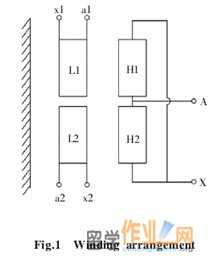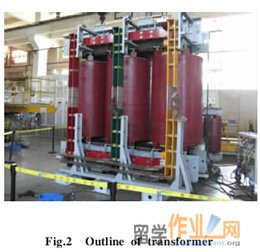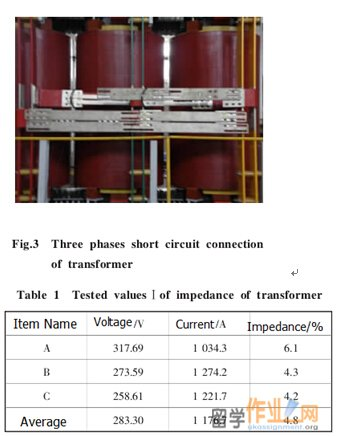# 英国纽卡斯尔大学留学生literature review范文

 1 Introduction 介绍   20MVA的变压器通常用于ITER交流电流测试平台到晶闸管的转换器提供实验功率。然而，由于ITER测试平台的大的系统阻抗，从而降低变压器阻抗的要求通常在实际变压器行业满足。本文将简要介绍如何变压器的阻抗降低至3％，以保证短路测试脉冲电流的要求。如何确保符合用户的测定值变压器阻抗要求，以确保基本平衡的关键是变压器设计的三相阻抗。The transformer of 20MVA is commonly used to ITER AC current testing platform to provide experimental power for thyristor converters. However, due to large system impedance of ITER testing platform, the requirement of reducing transformer impedance is commonly met in actual transformer industry. This paper will give a brief introduction of how to reduce transformer impedance to 3%, in order to ensure short-circuit test pulse current requirements. How to ensure compliance with the measured value transformer impedance requirements of users and to ensure basic balance is the key to the three-phase impedance of the transformer design.   2 The main technical parameters and load conditions of transformers变压器的主要技术参数和负载条件   2.1 The main technical parameters 与20MVA的额定容量，能够1000MVA下工作0.5秒，10kV的/0.4kV的额定电压，为50Hz，3％，与该三相阻抗不平衡率不超过10％的短路电阻的额定频率，加入群Yd11，并一一绝缘等级水平。   With rated capacity of 20MVA, able to work under 1000MVA for 0.5s, rated voltage of 10kV/0.4kV, rated frequency of 50Hz, short circuit resistance of 3 percent, with which the three-phase impedance unbalance rate does not exceed 10%, join group of Yd11, and insulation rating level of eleven.    2.2 Load conditions 提供交流电源至可控硅变换器，转换器的负载是感应性负载。验证短路故障条件下，直流侧的负载转换器的动态稳定的特点。变压器必须能够提供所需的短路电流，最大短路电流冲击，以350KA的冲击，短路试验时间为0.5s，不小于4h两个短电路测试时间间隔。Provide AC power to thyristor converters, converter load is an inductive load. Verify that the DC side load converter dynamic stability characteristics under short-circuit fault conditions. Transformer must be able to provide the required short-circuit the impact of current, the maximum short circuit current impact to 350kA, short circuit test time is 0.5s, two short circuit test intervals of not less than 4h.   3 Transformer impedance design calculations变压器阻抗设计计算   Due to the low short-circuit of the pulse transformer is with low voltage, and large electric current, so the key is of the transformer impedance design is to calculate transformer impedance and to estimate impedance of copper wire under low voltage.   3.1 Transformer impedance design calculations Considering the cost of transformer is highly influenced by the level of transformers impedance, the key is to design reasonable structure of low impedance transformer. There are three majormethods to reduce the two-winding transformer impedance as following. The first is to increase the core diameter was reduced a small number of turns increases the potential of winding turns. The second is to increase the electrical winding resistance that increases the height of the core high window. The third is split high and low voltage windings of the transformer impedance requirements of this article is only 3%, well below the normal capacity is 20MVA transformer impedance, not less than 8%. After considering the above three methods adopted by the special electromagnetic design optimization software to calculate and determine the impedance transformer body is 1.9%.   3.2 Impedance transformer low estimate of copper wire The low voltage transformer rated current up 28,867.5A, but this article requires closed delta connection. According to industry experience manufacturing low voltage high current transformer special "take the lead copper Low impedance transformer body impedance of 55%, that is 1.9 * 55% = 1.045%.   3.3 Calculation of the overall impedance transformer Whole body impedance transformer impedance and low-impedance copper wire, namely 1.9% + 1.045% = 2.95%.   4 Structural design of the transformer winding and lead变压器绕组和引线的结构设计   To meet the convenience transformer wiring and ensure that the transformer has a high mechanical strength and good resistance to shock pulse short circuit capacity, low-voltage wire winding and structural design of the transformer to take appropriate measures, as follows. 4.1 Transformer high voltage winding of the structural design The transformer high and low voltage windings were split into equal volume of two windings disposed axially down, similar to the structure of the axial double split, and through external leads connected in parallel as figure 1. Segmented cylindrical of high voltage winding, two parts winding around the system as a whole, the whole cast. Low uses foil winding, winding two parts were cast; using a special technology of suit does not make the top and bottom halves displacement between the windings.4.2 Structural design of low voltage copper wire Structure and low voltage transformer connection copper wire using the up and down two sets of low voltage windings connected to each triangle separately, then the corresponding phase by vertical copper connected in parallel to achieve "in the middle row of vertical copper leads to a three-phase outlet. This makes it easy lead copper solid and reliable support and positioning. Copper wire structure and connections, see figure 2.5 Transformer impedance measured data and analysis变压器阻抗测量数据及分析   After the completion of the transformer assembly of transformer impedance test, in the middle of a three-phase short vertical copper bars, the transformer is assembled as figure 3. Transformer impedance values measured figures are tested as Table 1.From table 1 the measured data, the measured impedance transformer value far exceeded the design value, up 4.8 percent, the average of the three-phase impedance serious imbalance, the maximum phase of 6.1%, 4.2% minimum phase. For this purpose, after preliminary analysis, that the main reason may be the following five aspects. The first is a three-phase transformer body impedance and impedance imbalance is too large. the second is a low voltage transformer winding two parts each implemented delta connection, and arranged in the upper and lower ends of the transformer leakage between nearby magnetic zone, copper windings and electromagnetic coupling, copper mutual inductance between the larger, increasing the fillet impedance copper itself. the third is a three-phase transformer copper fillet length and be relatively inconsistent ay short, while cx longer, resulting in a three-phase copper impedance imbalance. Fourth, low voltage transformer for each phase two vertical copper is actually the line current of each phase is divided into two branches, the two branch current of the same size, the same direction, alternating magnetic flux generated mutually reinforcing, while the two branches of the mutual inductance is large copper, increasing the vertical copper per phase impedance. Fifth is an impedance transformer short circuit test points Select the possible unreasonable. The above analysis has decided to carry out targeted impedance test trials, in order to find the product of serious impedance measured value is too large and the three-phase impedance based on the real reason for the serious imbalance.   6 Field trials using the method of discovery, analyze problems场试验采用发现法，分析问题   6.1 Impedance test transformer body Its purpose is the measured impedance transformer body is too large and the three-phase impedance is balanced! Specific operating angle \$ remove two parts connected to the low voltage winding of copper and three-phase vertical copper, head directly to the upper and lower part of the low voltage transformer windings Department shorted, see figure 4, the measured impedance values transformer body see table 2.